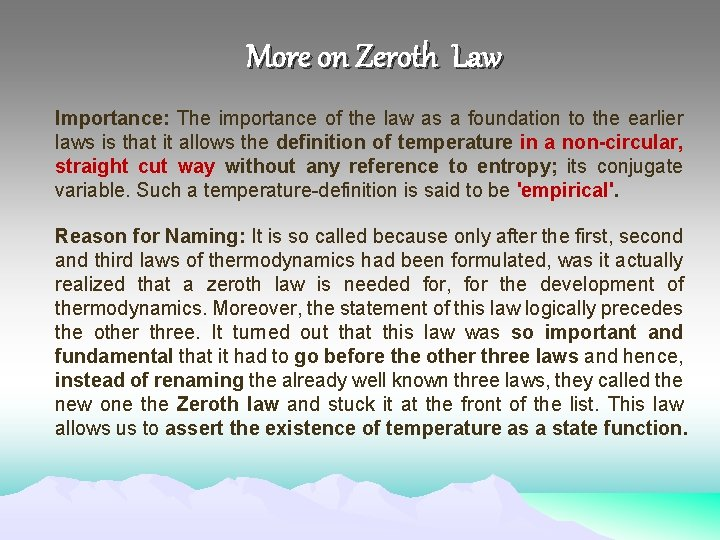CHEMISTRY PHYSICAL CHEMISTRY THERMODYNAMICS Lesson 3 by Dr

• Slides: 7CHEMISTRY PHYSICAL CHEMISTRY & THERMODYNAMICS Lesson 3 by Dr. Suchandra Chatterjee Department of Chemistry Surendranath CollegeLaws of Thermodynamics There are four laws of thermodynamics and they are some of the most important laws of nature. They were obtained from physical experiences from nature only and not from any laboratory experiments; hence very fundamental and can never be violated. So if anyone claims to have violating any of these laws, must be wrong anyhow. Sometimes fallacies are presented cleverly, but you must always remember your are actually being fooled in some way or other. The four thermodynamic laws actually define fundamental physical quantities (temperature, internal energy and entropy) that characterize thermodynamic systems. They also describe how these quantities or thermodynamic properties behave under various circumstances and forbid certain phenomena.Zeroth Law Statement: Two systems that are each found to be in thermal equilibrium with a third system will be found to be in thermal equilibrium with each other. Clarification: If you assume a water bucket in which you put a cup of tea, then if water is in thermal equilibrium with tea and tea is in thermal equilibrium with the surrounding, then you have to say that water is in thermal equilibrium with the surrounding as well. Basically, if A = B and C = B, then A = C must be. Note: This may seem so obvious that it doesn’t need stating but without this law we couldn’t ever define temperature and couldn’t build thermometers!More on Zeroth Law Importance: The importance of the law as a foundation to the earlier laws is that it allows the definition of temperature in a non-circular, straight cut way without any reference to entropy; its conjugate variable. Such a temperature-definition is said to be 'empirical'. Reason for Naming: It is so called because only after the first, second and third laws of thermodynamics had been formulated, was it actually realized that a zeroth law is needed for, for the development of thermodynamics. Moreover, the statement of this law logically precedes the other three. It turned out that this law was so important and fundamental that it had to go before the other three laws and hence, instead of renaming the already well known three laws, they called the new one the Zeroth law and stuck it at the front of the list. This law allows us to assert the existence of temperature as a state function.FIRST LAW Popular Statements: Energy can neither be created nor destroyed. It can only change its forms. In any process, the total energy of the universe remains the same. For a thermodynamic cycle the net heat supplied to the system equals the net work done by the system. Energy is always conserved; it can neither be created nor destroyed, just changed from one form to another, The total amount of energy in an isolated system remains conserved. The energy in a system can be converted to heat or work or other things, but you always have the same total that you started with. Energy of the universe always remains conserved. Perpetual motion machine of first kind (a machine that works for infinite time without supply of any external input energy) is impossible.More on first Law Analogy: Think of energy as indestructible blocks. If you have 30 blocks, then whatever you do to or with the blocks, you will always have 30 of them at the end. You can't destroy them, only move them around or divide them up, but there will always be 30. Sometimes you may lose one or more, but they still have to be taken account of because Energy is Conserved. Mathematical Statement: The increase in internal energy U of a closed system is equal to the heat Q supplied to the system minus work done W by it. d. U = d. Q-d. W. In other words, whatever amount of heat is given to the system, it is not fully converted into work. Some portion is utilized to perform the work, however, the residual amount does not go into waste, but remains stored in the system as internal energy U, so that, it can be used in future whenever situation demands, e. g. , in adiabatic processes, where system does work at the cost of internal energy stored in it!Thank you! ANY QUESTION ?